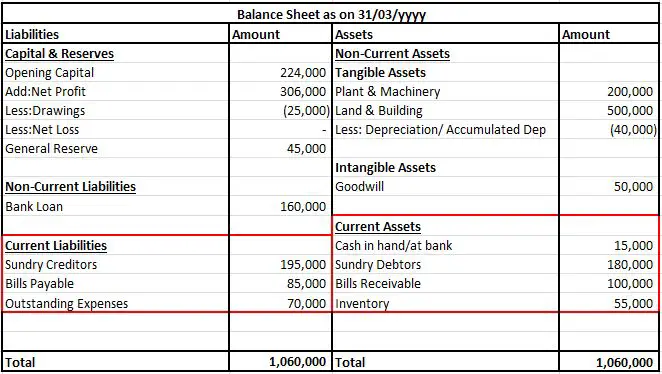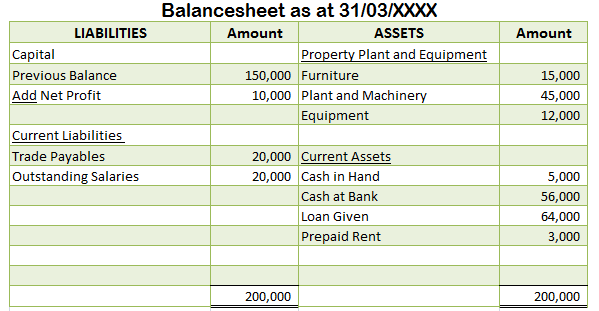## What is the meaning of negative working capital?

The term negative working capital is derived from the concept of working capital. To begin with, I would like to briefly explain the meaning of working capital. In simple terms, Working Capital refers to the total amount of current assets excluding the total amount of current liabilities in a busineRead more

The term negative working capital is derived from the concept of working capital. To begin with, I would like to briefly explain the meaning of working capital.

In simple terms, Working Capital refers to the total amount of current assets excluding the total amount of current liabilities in a business. It can have a positive or a negative value, wherein the two are an indicator of the well-being of a business. The formula to calculate working capital is as follows:

 WORKING CAPITAL  = TOTAL CURRENT ASSETS – TOTAL CURRENT LIABILITIES

# Negative Working Capital

In simple words, Negative working capital refers to the excess of net current liabilities over the net current assets. As the word itself suggests, a ‘negative’ working portrays a downfall in the financial position of a business and its inefficient functioning. A company is said to be facing financial difficulty and is not in a position to pay off its debts when the value of working capital is negative.

 NEGATIVE WORKING CAPITAL  = TOTAL CURRENT LIABILITIES > TOTAL CURRENT ASSETS

## EXAMPLE

Calculate the working capital of XYZ Ltd.

(Extract of Balance Sheet)

 PARTICULARS AMOUNT CURRENT ASSETS Cash and Cash Equivalents 36,000 Accounts Receivables 20,000 Stock Inventory 15,000 Marketable Securities 35,000 Prepaid Rent 7,000 TOTAL CURRENT ASSETS 1,13,000 CURRENT LIABILITIES Accounts Payable 15,000 Accrued Expense 4,000 Deferred Revenue 40,000 Taxes Payable 50,000 Short – Term Debt 10,000 Interest Payable 7,000 TOTAL CURRENT LIABILITIES 1,26,000

Note: As we can see the total current liabilities of XYZ Ltd. are exceeding the total current assets therefore, the working capital is negative.

Working capital = Total current assets – Total current liabilities

= 1,13,000 – 1,26,000

= (13,000)

Hope this helps.

See less
• 1
• Share
Share

## What is zero working capital?

Zero Working Capital Before diving into the concept of Zero Working Capital, let me help you understand the meaning of Working Capital. Working Capital is the term used to demonstrate whether the company possesses adequate current assets to discharge off its current liabilities. It is calculated asRead more

## Zero Working Capital

Before diving into the concept of Zero Working Capital, let me help you understand the meaning of Working Capital.

Working Capital is the term used to demonstrate whether the company possesses adequate current assets to discharge off its current liabilities. It is calculated as follows:

Working Capital = Total Current Assets – Total Current Liabilities

Now, taking this forward let us interpret the theory of Zero Working Capital.

The Working Capital of a company can be positive or negative, ie. the total current assets may exceed the total current liabilities or vice-versa. However, there can be a situation when the total current assets are equivalent to the total current liabilities of the company. Such a situation is referred to as Zero Working Capital. Zero Working Capital is when,

Total Current Assets = Total Current Liabilities, or

Total Current Assets – Total Current Liabilities = Zero

##Using the data given in the balance sheet above, let us calculate the zero working capital.

1. Total Current Assets = Cash in hand/bank + Sundry Debtors + Bills Receivable + Inventory
= 15,000 + 1,80,000 + 1,00,000 + 55,000
= 3,50,000

2. Total Current Liabilities = Sundry Creditors + Bills Payable + Outstanding Expenses
= 1,95,000 + 85,000 + 70,000
= 3,50,000

As there is no excess of Total Current Assets over the Total Current Liabilities, this situation is referred to as Zero Working Capital.

## Benefits and Approach of Zero Working Capital

Zero Working Capital is one of the latest techniques in working capital management. Let us now understand the benefits and approach of zero working capital in real-life scenario.

1. Reduction in the level of investments in working capital

Zero Working Capital is a strategy to reduce the level of investment in the working capital and thereby increase the investments in the long term assets. Following this strategy, companies avoid excess investments in current assets and prefer paying off their current liabilities using the existing current assets only.

2. Savings in Opportunity Cost of Funds

Working Capital earns a very low rate of return as compared to long term investments. Also, maintaining zero working capital will help save the opportunity cost of funds as the company can now use the excess funds to exploit various other opportunities.  So, owing to its benefits, the management would certainly prefer zero working capital.

3. Just-in-Time Methodology

Zero Working Capital approach will be possible only if the Just-in-Time methodology is adopted by the company. Following the demand-based production and distribution system is advised. Very low or zero inventory is emphasized. Everything should be produced and supplied as and when the demand for the same arises.

To keep in pace with the Just-in-Time practice, the receivable and payable terms should also be modified. Payable time granted by the supplier should be extended and the credit terms granted to the debtors should be cutback. This will ensure that you have the cash required to fund the supplier’s payment.

## Conclusion

Zero Working Capital eventually helps in better management of the current assets and current liabilities but still considered to be a difficult scenario to be implemented in practical business life.

See less
• 0
• Share
Share

## What is the formula for working capital?

Working capital = Current Assets - Current Liabilities I believe if you want to understand how the above formula works you will need to understand each part of this formula i.e you will first need to have a clear understanding about the concept of current assets and current liabilities. Current asseRead more

## Working capital = Current Assets – Current Liabilities

I believe if you want to understand how the above formula works you will need to understand each part of this formula i.e you will first need to have a clear understanding about the concept of current assets and current liabilities.

Current assets

It refers to all the assets including cash and cash equivalents which are expected to be converted within a year or within the operating cycle of an entity if such entity has an operating cycle longer than a year.

Current liabilities

It refers to all the payables or debts which an entity expects to discharge within a year or the operating cycle of such entity provided such an entity has an operating cycle longer than a year.

I understand that even though you got a rough idea of this concept you may not be confident while applying this concept hence, to relate to this concept a numerical example would be of great help.

So, I believe once you have a look at the below-mentioned example you will be in a better position to comfortably apply this formula:Here, Working Capital = Current Assets – Current Liabilities

= Cash in Hand + Cash at Bank + Trade Receivables + Prepaid Rent –Trade Payables  –                  Outstanding Salaries

= 5,000 + 56,000 + 64,000 + 3,000 – 20,000 – 20,000

= 128,000 – 40,000

= 88,000.

It will add more value to your understanding if you also interpret the concept of Gross Working Capital and Net Working Capital.

Gross Working Capital

Gross working capital is a sum total of current assets of an entity. It includes

• Cash and Cash Equivalents
• Inventory
• Short term Investments
• Other Marketable Securities.

Net Working Capital

Now, the net working capital of an entity is nothing but the working capital of an entity. In simple terms, it is a difference between current assets and current liabilities of an entity.

Aastha Mehta

See less
Attachment

• 0
• Share
Share